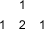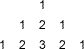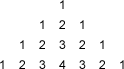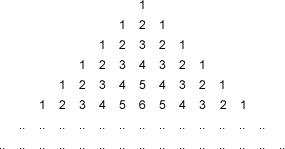#### You may also like### Archimedes and Numerical Roots

The problem is how did Archimedes calculate the lengths of the sides of the polygons which needed him to be able to calculate square roots?### Rationals Between...

What fractions can you find between the square roots of 65 and 67?### Root to Poly

Find the polynomial p(x) with integer coefficients such that one solution of the equation p(x)=0 is $1+\sqrt 2+\sqrt 3$.

# Lost in Space

##### Age 14 to 16 Challenge Level:

There are three ways to enter the triangular array of numbers in the diagram below, starting with 1, and counting from 1 -2 in order, finishing with the two in the bottom row.How many ways are there to count 1 - 2 - 3 in the following array?And 1 - 2- 3 - 4 on this?Can you generalize?Can you explain what you find?